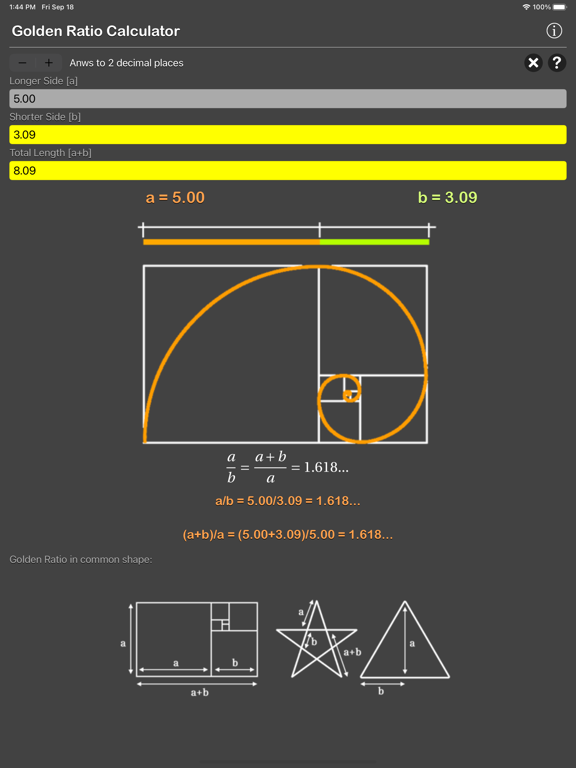クイックアプリ検索 »   新着 | 値下げ | 無料 | 高評価 | 新着オススメ！ | キニナル！ | 新着動画 | iPad新着 | iPad値下げ | iPadオススメ！
APPLICATION INFO - iPhoneアプリの詳細情報
TOP > ユーティリティ > Golden Ratio Calculator Plus# Golden Ratio Calculator Plus

¥370
The Golden Ratio Calculator are physic/math calculator to calculate the longer side [a] , shorter side[b] or combined length of the two sides [a+b] to compute the golden ratio.

Features:
- Instant calculation
- Result are copy able to other app
- Formula are include as reference
- Support up to 16 decimal place
- Support various unit for each input

The golden ratio φ (symbol is the Greek letter "phi")
is a special number approximately equal to 1.618

In mathematics, two quantities are in the golden ratio if their ratio is the same as the ratio of their sum to the larger of the two quantities. The figure on the right illustrates the geometric relationship.
The golden ratio, also known as the golden mean, is the value phi where phi = (A+B)/A = A/B.

The golden ratio is an irrational mathematical constant.
φ phi = 1.6180339887

The Golden Ratio is also sometimes called the golden section, golden mean, golden number, divine proportion, divine section and golden proportion.
It appears many times in geometry, art, architecture and other areas. As Golden Ratio makes the most pleasing and beautiful shape.

Thanks for your support and do visit nitrio.com for more apps for your iOS devices.このアプリはiPhone、iPadの両方に対応しています。
カテゴリー
ユーティリティ
リリース
2018/10/28

2020/9/18
バージョン
1.1

サイズ
8.3 MB

このバージョンの新機能
- Bug Fixed.
••••••••••